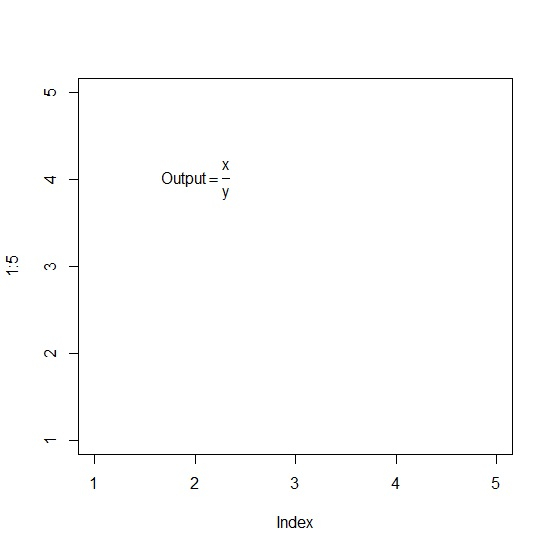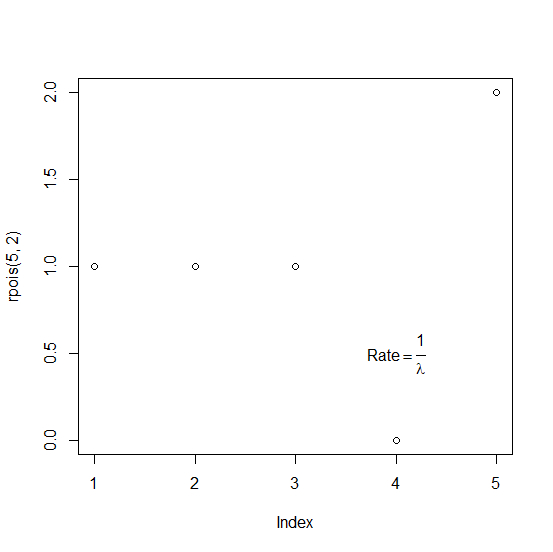# How to display fraction in base R plot?

To display fraction in base R plot, we can use frac function in text function.

For example, if we create a plot of vectors called X and Y with display of over form fraction as X over Y at X=2 and Y= 2 then we can use the following code −

text(2,2,expression(Output==frac(X,Y)))

Check out the below examples to understand how it works.

## Example 1

To display fraction in base R plot, use the code given below −

plot(1:5,type="n")
text(2,4,expression(Output==frac(x,y)))

## Output

If you execute the above given code, it generates the following output −## Example 2

To display fraction in base R plot, use the code given below −

plot(rpois(5,2))
text(4,0.5,expression(Rate==frac(1,lambda)))

## Output

If you execute the above given code, it generates the following output −